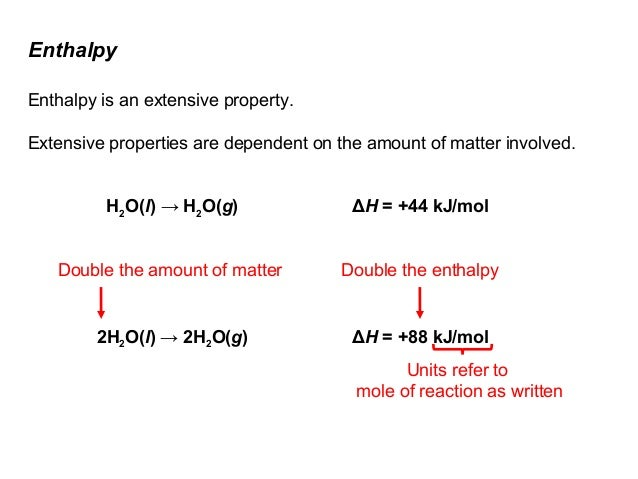# Why is enthalpy an extensive property?

Jul 19, 2014

First, an extensive property is one that depends on the amount of material present. For example, mass is an extensive property because if you double the amount of material, the mass doubles. An intensive property is one that does not depend on the amount of material present. Examples of intensive properties are temperature $T$ and pressure $P$.

Enthalpy is a measure of heat content, so the greater the mass of any substance, the greater the amount of heat that it can hold at any particular temperature and pressure.

Technically, enthalpy is defined as the integral of the heat capacity at constant pressure from absolute zero to the temperature of interest, including any phase changes. For example,

$\Delta H = {\int}_{{T}_{0 K}}^{{T}_{\text{goal}}} {C}_{P} \mathrm{dT}$

$= {\int}_{{T}_{0 K}}^{{T}_{\text{fus") C_PdT + DeltaH_"fus" + int_(T_"fus")^(T_"vap") C_PdT + DeltaH_"vap" + int_(T_"vap")^(T_"goal}}} {C}_{P} \mathrm{dT}$

if we suppose that the temperature of interest is above the boiling point. Then, we go through ${T}_{0 K} \to {T}_{\text{fus" -> T_"vap" -> T_"goal}}$.

If two samples are identical at the same temperature and pressure, except that Sample B has twice the mass of Sample A, then the enthalpy of Sample B is twice that of Sample A.

That's why enthalpy values are usually quoted as J/mol or kJ/mol. If you multiply the quoted value by the number of moles of substance, you get the enthalpy in J or kJ.May 10, 2018

Enthalpy by definition (units of J) is an extensive property as it proportional to the amount of the components in the system at hand. However, it is also an intensive property when quoted in kJ/mol or kJ/kg.

#### Explanation:

Enthalpy, $H$, is defined as

$H = U + p V$

$U = \text{internal energy}$
$p = \text{pressure}$
$V = \text{volume}$

However, we can't directly measure the total enthalpy of a system, so we can only measure changes in enthalpy.

A change in enthalpy is the heat evolved or absorbed at constant pressure in a specific reaction/process.

This change in enthalpy at constant pressure is now given by

∆H = ∆U + p∆V

The SI unit for an enthalpy change is the joule (J), and it depends on how much of the components in the system you have. The more of the substance(s) you have, the more heat can be absorbed or released for a given change. For example, vaporising 100 g of water takes double the amount of energy as the same process for 50 g of water. This makes enthalpy an extensive property.

However, tables of enthalpy values are commonly quoted as molar enthalpy (kJ/mol) and specific enthalpy (kJ/kg). These are intensive properties as they already take into account the amount of the components (one mole or one kg).

There are several different types of enthalpy changes such as phase changes, enthalpies of reaction and so forth. They could be given in kJ or kJ/mol. Which one dictates whether it is an intensive or extensive property.

Here is my rationale by way of an example and an analogy. Note that we are using kJ instead of J, as that is what is commonly used.

To vaporise one mole of water at 298 K

∆H = 44" kJ"

or

∆H_"vap"(H_2O) = 44" kJ/mol"

These two quantities are related by the expression

∆H_"vap"(H_2O) = (∆H)/n

The enthalpy change (∆H) is extensive, whereas the molar enthalpy of vaporisation (∆H_"vap"(H_2O)) is intensive.

Now let's have a look at density, which is an intensive property. The following two equations are comparable

$\text{density"="mass"/"volume}$

and

∆H_"vap"(H_2O) = (∆H)/n

The change in enthalpy for a certain amount (n) is given in kJ by

∆H = ∆H_"vap"(H_2O)*n

just as the mass in a given volume of substance is given by

$\text{mass"="density"*"volume}$

So you see

$\text{density"-=∆H_"vap} \left({H}_{2} O\right)$

"mass"-=∆H

$\text{density}$ and ∆H_"vap"(H_2O) are intensive, whereas $\text{mass}$ and ∆H are extensive.